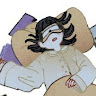Search
•Lena Du

Updated: Apr 21

## 1. Brief description

This project is using image blending to combine contents from 2 different images. The idea is to construct a linear system, which is restricted by the gradient source image and the target image, according to:

v=argminv∑i∈S,j∈Ni∩S((vi−vj)−(si−sj))2+∑i∈S,j∈Ni∩¬S((vi−tj)−(si−sj))2

The problem can be simplified to:

Av=b

and our target is to construct A and b, therefore solve v. Therefore, v should correspond to the area that we are concerned with, which means that we need to represent the area as a vector, and then transform it into an image. Since we are dealing with colorful images, we should process R, G, B, channels separately. Each channel is corresponding to a 2D array that represents the brightness at each pixel, we can then flatten this 2D array to a vector that has height * width elements. Correspondingly, we can transform the vector to the image by reshaping it. We construct 3 pairs of A and B, obtain 3 v for each channel, reshape each channel, and stack them into one completed image.

## 2. Problem Completion

### 2.1 Toy Problem

The toy problem introduced the easiest way to construct a linear system to solve the vector v. The number of rows in A corresponds to the number of restrictions. In this case, we consider two directions of each pixel, with the boundary condition, there are 2 * (height - 1) * (width - 1) rows in matrix A. Since the v is the flattened vector of the grayscale image we want to obtain, its length is naturally height * width as the number of pixels in the original image. The output is the same as the input.### 2.2 Poisson Blending

It is also intuitive to implement the solution based on Ax = b for Poisson Blending. The objective is to blend a part of one image into another. Since the edge is unnatural and needed to be blended, we need to use both information from the source image (to be pasted) and the target image (background).

In this case, we are going to construct A with 4 directions of each pixel. Therefore, each pixel (except boundaries like right edge and bottom edge) corresponds to 4 rows in A, which would be 4 * (height * length) in total. If we consider v as the flattened image of each channel, then we need A to have height * width columns. This will be potentially redundant: what if we only blend a small area? If we use this method, we still need to resolve a large linear system even we are only concerned about a small part.

Thus, we can obtain v as the area that we are only concerned about. Then the length of v equals the number of pixels of the area to be blended, so does the number of columns in A. This is more rational and reduces both time and space complexity. We only need to remap the value in v back to its corresponding position after calculating the linear system.

Poisson Blending considers the edge of the pasted area and makes the combination more natural, especially around the edge. However, for some large source image to be blended, pixels near around the center of the source image is not as good as those around the edges. For example, if we want to blend some image into a textured surface, the result with Poisson Blending loses the texture information while we actually need it. There are more examples in section 3.

Therefore, we can change the objective to:

v=argminv∑i∈S,j∈Ni∩S((vi−vj)−dij)2+∑i∈S,j∈Ni∩¬S((vi−tj)−dij)2

where

dij=si−sj,ifabs(si−sj)>=abs(ti−tj),dij=ti−tj,ifabs(si−sj)<abs(ti−tj).

This helps to maintain the key information from both the source image and the target image.

## 3. Results

### 3.1 Favorite blending result

For the first one, this is a nice way to preview if a design shows great on a certain product. This has potential business value and I am satisfied with it. For the second one, in the advertisement industry, it is common to use special effects to make food photos, and this could be very useful for their objective. For the third one, I like how it looks like to put a tattoo onto a piece of textured paper. Also, we can reverse this process to show if a painting shows great as a tattoo, and help the customer to decide if they are going to do it.

### 3.2 Supplemental results

This shows the difference between Poisson Blending and Mixed Blending. The result with Poisson Blending loses the texture information while we actually need it, but the Mixed result well shows the texture.